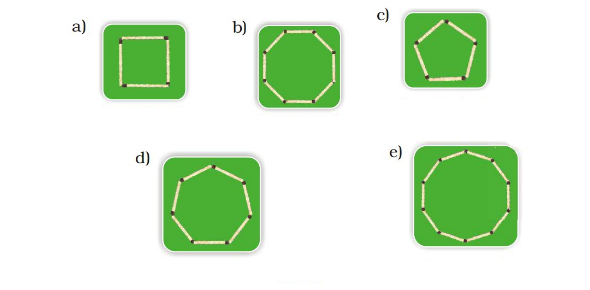# Shapes And Angles Test Quiz!

10 Questions | Total Attempts: 324Settings.

• 1.
Please select the clock with the right angle.
• 2.
Select the clock with the Obtuse angle.
• 3.
Which shapes angle does not change when pressed?
• 4.
The angle marked in [Blank] color is the biggest angle.
• 5.
Are the angles marked with yellow equal true or false?
• A.

True

• B.

False

• 6.
An angle whose measure is between 0˚ and 90˚ is called an __________________ angle.
• A.

Obtuse Angle

• B.

Acute Angle

• C.

Right Angle

• D.

Straigth Angle

• 7.
What kind of angle it is?
• A.

90 Degree angle

• B.

More than 92 degree angle

• C.

Less than 90 degree angle

• D.

180 degree angle

• 8.
What measure (in degrees) should be added to 34-degree angle to make a right angle?
• A.

55 degree

• B.

56 degree

• C.

66 degree

• D.

40 degree

• 9.
What are the angles between two hands of a clock at 2 O’ clock?
• A.

Right Angle

• B.

Acute angle

• C.

Obtuse Angle

• D.

Straight angle

• 10.
Angles can be measured using a [Blank]
Related TopicsBack to top